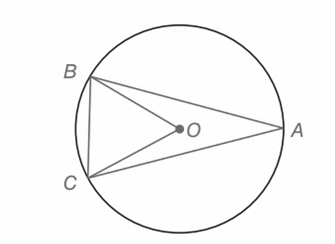Chapter 6.CT, Problem 3CT### Elementary Geometry for College St...

6th Edition
Daniel C. Alexander + 1 other
ISBN: 9781285195698

#### Solutions

Chapter
Section### Elementary Geometry for College St...

6th Edition
Daniel C. Alexander + 1 other
ISBN: 9781285195698
Textbook Problem
4 views

# a) If m ∠ B A C = 24 ° , then m B C ⌢ = _____________.b) If A B ⌢ ≅ A C ⌢ , then Δ A B C is a(n)___________ triangle.To determine

(a)

To find:

The measure of mBOC

Explanation

Given:

mBAC=24°

Approach:

1) In a circle the measure of an arc is twice the measure of the interior angle that intercepts the arc.

2) In a circle if two arcs are congruent, then the chord that intercepts the arcs are congruent.

Calculation:

Consider the given circle,

mBAC=24°.

BC is the arc that intercept the interior angle

To determine

(b)

To find:

The measure of mBAC

### Still sussing out bartleby?

Check out a sample textbook solution.

See a sample solution

#### The Solution to Your Study Problems

Bartleby provides explanations to thousands of textbook problems written by our experts, many with advanced degrees!

Get Started